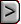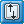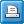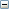﻿ NWT - Newton Solver
Online Guide to MODFLOW-NWT

NWT - Newton SolverNWT - Newton SolverNWT - Newton SolverAbbreviation in Name file

NWT

Purpose

The Newton solver is used to solve the finite difference equations in each step of a MODFLOW-NWT stress period.

Documentation

Niswonger, R.G., Panday, Sorab, and Ibaraki, Motomu, 2011, MODFLOW-NWT, A Newton formulation for MODFLOW-2005: U.S. Geological Survey Techniques and Methods 6-A37, 44 p.

release.txt for MODFLOW-NWT

Related Packages

Solvers

The NWT solver can only be used with the Upstream Weighting (UPW) package

Supported in

 • MODFLOW-NWT
 • MODFLOW-OWHM

Other Notes

 • All numeric variables are free format if the option “FREE” is specified in the Basic Package input file; otherwise, all variables have 10-character fields. Values in brackets are specified only for some input options. If the OPTIONS keyword is specified as “SIMPLE,” “MODERATE,” or “COMPLEX,” then all solver input variables following OPTIONS in item 1 are not required to be specified and are set to default values. If OPTIONS is specified as “SPECIFIED,” then all input variables are required; however, residual-control variables are only required if BACKFLAG is set to one. The residual-control option can only be used if OPTIONS is specified as “SPECIFIED.” It is recommended that users refer to table 2 and the discussion on “Example Input for the NWT Input File” for guidance on values that might be identified for each input variable.
 • The optional variable PHIRAMP in the Well package can be specified in MODFLOW-NWT.
 • If the Lake package is used, more iterations may be required.

Input to the Newton (NWT) Solver Package is read from the file that is type “NWT” in the Name File.

For Each Simulation

Data Set 0.

[#Text]

Item 0 is optional—“#” must be in column 1. Item 0 can be repeated multiple times.Text
 Text is a character variable (199 characters) that starts in column 2. Any characters can be included in Text. The “#”character must be in column 1. Lines beginning with # are restricted to the first lines of the file. Text is written to the Listing File.

Data Set 1.

HEADTOL FLUXTOL MAXITEROUT THICKFACT LINMETH IPRNWT IBOTAV OPTIONS [DBDTHETA] [DBDKAPPA] [DBDGAMMA] [MOMFACT] [BACKFLAG] [MAXBACKITER] [BACKTOL] [BACKREDUCE]Explanation of variablesHEADTOL
 HEADTOL (units of length)—is the maximum head change between outer iterations for solution of the nonlinear problem (real).FLUXTOL
 FLUXTOL (units of length cubed per time)—is the maximum root-mean-squared flux difference between outer iterations for solution of the nonlinear problem (real).MAXITEROUT
 MAXITEROUT is the maximum number of iterations to be allowed for solution of the outer (nonlinear) problem (integer).THICKFACT
 THICKFACT is the portion of the cell thickness (length) used for smoothly adjusting storage and conductance coefficients to zero (symbol Ω in equation 9; real).LINMETH

LINMETH is a flag that determines which matrix solver will be used (integer).

 • A value of 1 indicates GMRES will be used
 • A value of 2 indicates χMD will be used. The χMD can not be used with local grid refinement in MODFLOW-OWHM.IPRNWT
 IPRNWT is a flag that indicates whether additional information about solver convergence will be printed to the main listing file (integer).IBOTAV
 IBOTAV is a flag that indicates whether corrections will be made to groundwater head relative to the cell-bottom altitude if the cell is surrounded by dewatered cells (integer). A value of 1 indicates that a correction will be made and a value of 0 indicates no correction will be made. This input variable is problem specific and both options (IBOTAV=0 or 1) should be tested. MODFLOW-NWT provides two different methods for simulating dry cells in the deepest layer, and the option is defined by the NWT input file variable IBOTAV. Head for cells in the deepest layer, and for single-layer models, cannot fall below the cell-bottom altitude if the input variable IBOTAV is set to 1; otherwise, heads in the deepest layer can fall below the cell-bottom altitude. The value of IBOTAV does not affect the solution because dry bottom-layer cells are effectively ignored in the solution (that is, for a cell that does not receive inflow, the coefficients are essentially zero in the row of the matrix in which a bottom-layer cell is the diagonal element). IBOTAV is provided because it can affect convergence behavior for a cell with thin saturated thickness. The value of IBOTAV that provides the fastest convergence rate appears to be problem specific.OPTIONS

OPTIONS are keywords that activate options:

One and only one of the following four options must be specified.

 • SPECIFIED indicates that the optional solver input values listed for items 1 and 2 will be specified in the NWT input file by the user.
 • SIMPLE indicates that default solver input values will be defined that work well for nearly linear models. This would be used for models that do not include nonlinear stress packages, and models that are either confined or consist of a single unconfined layer that is thick enough to contain the water table within a single layer. (See table 2 for the solver input values that will be used for this option.)
 • MODERATE indicates that default solver input values will be defined that work well for moderately nonlinear models. This would be used for models that include nonlinear stress packages, and models that consist of one or more unconfined layers. The “MODERATE” option should be used when the “SIMPLE” option does not result in successful convergence. (See table 2 for the solver input values that will be used for this option.)
 • COMPLEX indicates that default solver input values will be defined that work well for highly nonlinear models. This would be used for models that include nonlinear stress packages, and models that consist of one or more unconfined layers representing complex geology and sw/gw interaction. The “COMPLEX” option should be used when the “MODERATE” option does not result in successful convergence. (See table 2 for the solver input values that will be used for this option.)

The following option may be specified.

 • CONTINUE For this input option, if the model fails to converge during a time step then it will continue to solve the following time step. However, if the "CONTINUE" is removed from this line then the model will stop after convergence failure. See also: the STOPERROR option in the Basic Package

Read the following values if OPTIONS = “SPECIFIED.”DBDTHETA
 DBDTHETA is a coefficient used to reduce the weight applied to the head change between nonlinear iterations (symbol θ in equation 21). DBDTHETA is used to control oscillations in head. Values range between 0.0 and 1.0, and larger values increase the weight (decrease under-relaxation) applied to the head change (real).DBDKAPPA
 DBDKAPPA is a coefficient used to increase the weight applied to the head change between nonlinear iterations (symbol κ in equation 22). DBDKAPPA is used to control oscillations in head. Values range between 0.0 and 1.0, and larger values increase the weight applied to the head change (real).DBDGAMMA
 DBDGAMMA is a factor (symbol γ in equation 19) used to weight the head change for iterations n–1 and n. Values range between 0.0 and 1.0, and greater values apply more weight to the head change calculated during iteration n (real).MOMFACT
 MOMFACT is the momentum coefficient m of equation 20 and ranges between 0.0 and 1.0. Greater values apply more weight to the head change for iteration n (real).BACKFLAG
 BACKFLAG is a flag used to specify whether residual control will be used. A value of 1 indicates that residual control is active and a value of 0 indicates residual control is inactive (integer).MAXBACKITER
 MAXBACKITER is the maximum number of reductions (backtracks) in the head change between nonlinear iterations (integer). A value between 10 and 50 works well. MAXBACKITER is only read if BACKFLAG > 0.BACKTOL
 BACKTOL is the proportional decrease in the root-mean-squared error of the groundwater-flow equation used to determine if residual control is required at the end of a nonlinear iteration, as applied in equation 23 (real). BACKTOL is only read if BACKFLAG > 0.BACKREDUCE
 BACKREDUCE is a reduction factor (symbol Br in equation 23) used for residual control that reduces the head change between nonlinear iterations (real). Values should be between 0.0 and 1.0, where smaller values result in smaller head-change values. BACKREDUCE is only read if BACKFLAG > 0.

If LINMETH = 1 and OPTIONS is set to “SPECIFIED” read the following line:

Data Set 2a.

[MAXITINNER] [ILUMETHOD][LEVFILL][STOPTOL][MSDR]Explanation of variablesMAXITINNER
 MAXITINNER is the maximum number of iterations for the linear solution (integer).ILUMETHOD

ILUMETHOD is the index for selection of the method for incomplete factorization (ILU) used as a preconditioner. See Kipp and others (2008) for further details (integer).

 • ILUMETHOD=1—ILU with drop tolerance and fill limit. Fill-in terms less than drop tolerance times the diagonal are discarded. The number of fill-in terms in each row of L and U is limited to the fill limit. The fill-limit largest elements are kept in the L and U factors.
 • ILUMETHOD=2 — ILU(k), Order k incomplete LU factorization. Fill-in terms of higher order than k in the factorization are discarded.LEVFILL
 LEVFILL is the fill limit for ILUMETHOD = 1 and is the level of fill for ILUMETHOD = 2. Recommended values: 5-10 for method 1, 0-2 for method 2. See Kipp and others (2008) for further details (integer).STOPTOL
 STOPTOL is the tolerance for convergence of the linear solver. This is the residual of the linear equations scaled by the norm of the root mean squared error. Usually 10-8 to 10-12 works well. See Kipp and others (2008) for further details (integer).MSDR
 MSDR is the number of iterations between restarts of the GMRES Solver. See Kipp and others (2008) for further details (integer).

If LINMETH = 2 and OPTIONS is set to “SPECIFIED” read the following line:

Data Set 2b.

[IACL][NORDER][LEVEL][NORTH][IREDSYS][RRCTOLS][IDROPTOL][EPSRN] [HCLOSEXMD][MXITERXMD]Explanation of variablesIACL

IACL is a flag for the acceleration method:

 • 1 = ORTHOMIN;
 • 2 = Bi-CGSTAB

(integer).NORDER

NORDER is a flag for the scheme of ordering the unknowns:

 • 0= original ordering;
 • 1= RCM ordering;
 • 2= Minimum Degree ordering

(integer).LEVEL
 LEVEL is the level of fill for incomplete LU factorization (integer).NORTH
 NORTH is the number of orthogonalization for the ORTHOMIN acceleration scheme. A number between 4 and 10 is appropriate. Small values require less storage but more iterations may be required. This number should equal 2 for the other acceleration methods (integer).IREDSYS

IREDSYS is a flag for reduced system preconditioning (integer):

 • 0=do not apply reduced system preconditioning
 • 1 =apply reduced system preconditioning;RRCTOLS
 RRCTOLS is the residual reduction-convergence criteria (real).IDROPTOL

IDROPTOL is a flag for using drop tolerance in the preconditioning (integer).

 • 0 = don't use drop tolerance
 • 1 = use drop toleranceEPSRN
 EPSRN is the drop tolerance for preconditioning (real).HCLOSEXMD
 HCLOSEXMD is the head closure criteria for inner (linear) iterations (real).MXITERXMD
 MXITERXMD is the maximum number of iterations for the linear solution.